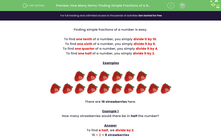# Find Simple Fractions of a Number

In this worksheet, students will find simple fractions of a number of items by dividing.Key stage:  KS 2

Curriculum topic:   Number: Fractions

Curriculum subtopic:   Use Fractions

Difficulty level:#### Worksheet Overview

Finding simple fractions of a number is easy.

To find one tenth of a number, you simply divide it by 10.

To find one sixth of a number, you simply divide it by 6.

To find one quarter of a number, you simply divide it by 4.

To find one half of a number, you simply divide it by 2.

ExamplesThere are 16 strawberries here.

Example 1

What would half of those strawberries be?

To find a half, we divide by 2.

16 ÷ 2 = 8 strawberries

Example 2

What would one eighth of those strawberries be?

To find one eighth, we divide by 8.

16 ÷ 8 = 2 strawberries

Example 3

How many strawberries would there be in a quarter of the number?

To find one quarter, we divide by 4.

16 ÷ 4 = 4 strawberries

Are you happy with all that?Want a bit more help with this before you begin? Why not watch this short video?

### What is EdPlace?

We're your National Curriculum aligned online education content provider helping each child succeed in English, maths and science from year 1 to GCSE. With an EdPlace account you’ll be able to track and measure progress, helping each child achieve their best. We build confidence and attainment by personalising each child’s learning at a level that suits them.

Get started